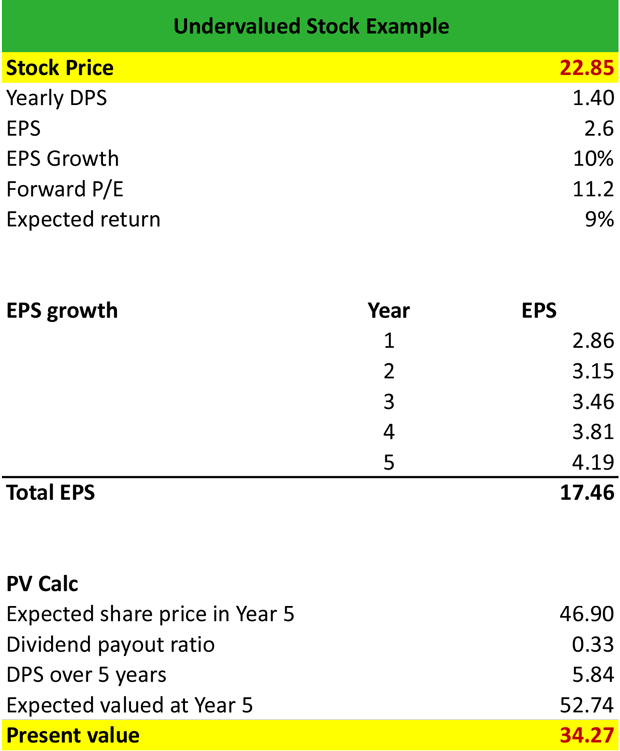# What are Undervalued Stocks?

Definition: Undervalued stocks are securities that trades lower than its fair market value, i.e. the value that the company’s cash flow and return on assets justify. Undervalued securities are expected to increase rapidly, and make up for good “buy” opportunities.

## What Does Undervalued Stocks Mean?

What is the definition of undervalued stocks? Undervalued securities have a market price that is significantly lower than their fair value (market value < fair value) as a result of decreasing investor confidence or consensus estimates. Financial analysts use the price-to-earnings ratio (P/E) or calculate the growth rate of a firm to determine if a stockis undervalued.

Stocks are deemed as undervalued either following a decline in demand driven by declining investor confidence or if the firm’s fundamentals improve rapidly while the market price remains constant. In both cases, if the company’s fundamentals and the analyst growth projections do not justify a decline in the market price, the stock is possibly undervalued.

Let’s look at an example.

## Example

Alexis is an investment advisor, and he manages a client’s portfolio. He suspects that at least one of the stocks in the portfolio is trading lower than its fair value. So, he decides to calculate the fair value of the stock.

The stock currently trades at \$22.85, declares an annual dividend per share of \$1.40, and has an EPS of \$2.60. Alexis assumes an EPS growth of 10% for the next 5 years annually, a forward P/E of 11.2 and an expected return of 9%.

First, he calculates the total EPS for the next 5 years as follows:

Year 1 = EPS in Year 0 x (1 + EPS growth) = \$2.60 x (1+10%) = \$2.86

Year 2 = EPS in Year 1 x (1 + EPS growth) = \$2.86 x (1+10%) = \$3.15

Year 3 = EPS in Year 2 x (1 + EPS growth) = \$3.15 x (1+10%) = \$3.46

Year 4 = EPS in Year 3 x (1 + EPS growth) = \$3.46 x (1+10%) =\$ 3.81

Year 5 = EPS in Year 4 x (1 + EPS growth) = \$3.81 x (1+10%) = \$4.19

The total EPS for the next 5 years is \$2.86 + \$3.15 + \$3.46 + \$3.91 + \$4.19 = \$17.46

Then, he calculates the expected value of the stock in Year 5 as follows:

Expected share price in year 5 = EPS in year 5 x Forward P/E = \$4.19 x 11.2 = \$46.90

Dividend payout ratio = DPS / EPS in year 5 = \$1.40 / \$4.19 = 0.33

Dividend per share in Year 5 = Dividend payout ratio x total EPS = 0.33 x \$17.46 = \$5.84

Expected value at Year 5 = Expected share price in year 5+ Dividend per share in Year 5 = \$46.90 + \$5.84 = \$52.74Then, he discounts the present value of the stock by the expected return of 9% = \$52.74 x (1+9%) ^5 = \$34.27

Since the stock currently trades at \$22.85 and its fair value is \$34.27, i.e. market value < fair value = the stock is undervalued.

## Summary Definition

Define Undervalued Stocks: Under-valued stock means a security or share that is trading for less than the actual price it is worth.